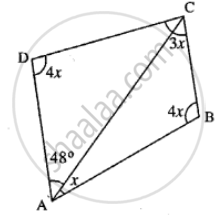# From the Following Figure Find ; (I) X (Ii) ∠Abc (Iii) ∠Acd - Mathematics

Sum

From the following figure find ;
(i) x
(ii) ∠ABC
(iii) ∠ACD

#### Solutionx + 4x + 3x + 4x + 48° = 360°

12x = 360° – 48°

12x = 312

x = 312/12 = 26°

(ii) ∠ABC = 4x

4 × 26 = 104°

(iii) ∠ACD = 180° - 4x - 48°

= 180° - 4 × 26° - 48°

= 180° - 104° - 48°

= 180° - 152° = 28°

Is there an error in this question or solution?
Chapter 16: Understanding Shapes - Exercise 16 (C) [Page 187]

#### APPEARS IN

Selina Concise Mathematics Class 8 ICSE
Chapter 16 Understanding Shapes
Exercise 16 (C) | Q 6 | Page 187
Selina Class 6 Mathematics